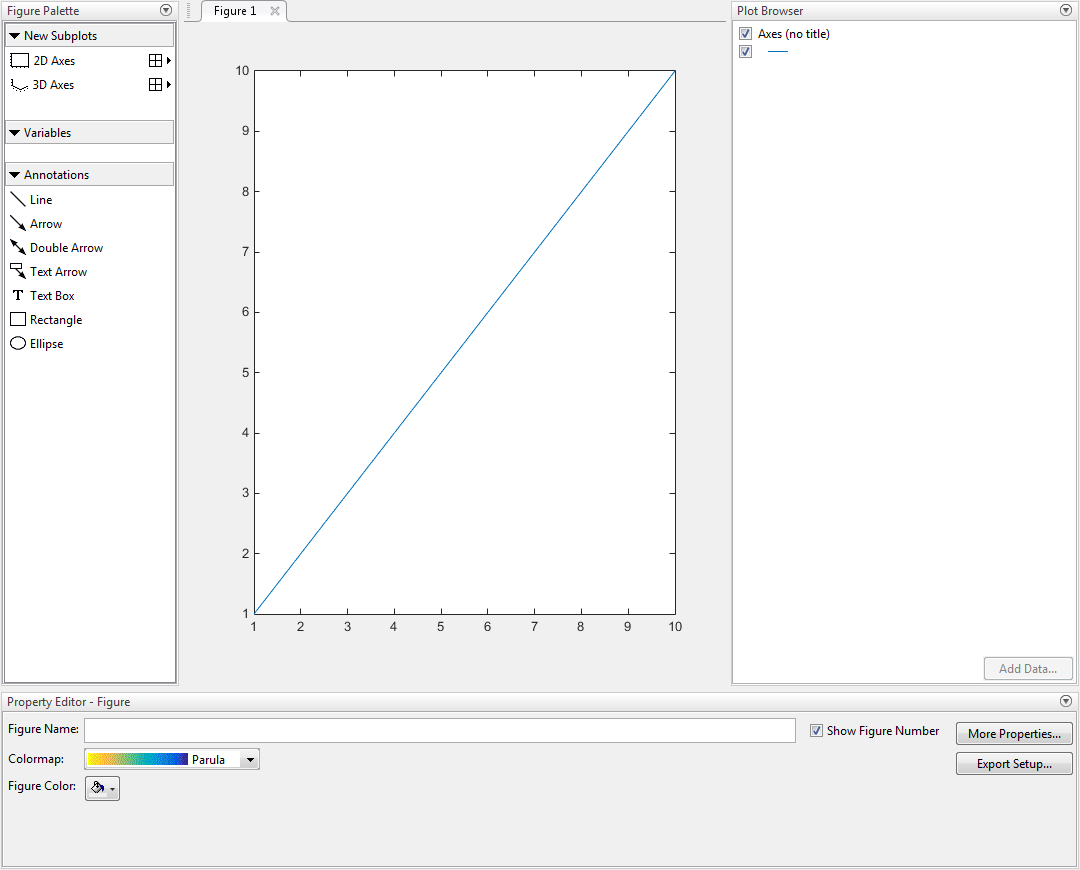# plottools

(Not recommended) Show or hide plot tools

`plottools` is not recommended. Use `inspect` to launch the Property Inspector instead. For more information, see Compatibility Considerations.

## Syntax

``plottools``
``plottools(state)``
``plottools(state,tool)``
``plottools(fig,___)``

## Description

````plottools` shows the plot tools on the current figure. If no current figure exists, `plottools` will create one.The first time you open the plot tools, the Figure Palette, Plot Browser, and Property Editor appear, grouped around the current figure. If you close, move, or undock any of the tools, MATLAB® restores that configuration when you invoke the tools for subsequent figures, both within and across MATLAB sessions.```
````plottools(state)` changes the state of the plot tools on the figure. For example, `plottools("on")` shows the plot tools.```
````plottools(state,tool)` operates on the specified tool only. For example, `plottools("on","figurepalette")` shows the Figure Palette but not the Plot Browser or Property Editor.```

example

````plottools(fig,___)`operates on the specified figure instead of the current figure. The input `fig` can precede any of the input argument combinations in the previous syntaxes.```

## Examples

collapse all

Show the plot tools on a figure by calling the `plottools` function. The tools open in the same configuration as last time you invoked the tools.

```f = figure plot(1:10); plottools(f)```## Input Arguments

collapse all

Plot tool state, specified as one of these values:

• `"on"` — Show plot tools.

• `"off"` — Hide plot tools.

• `"toggle"` — Switch the plot tools state between the `"on"` and `"off"` states.

Example: `plottools("on")` shows the plot tools on the current figure.

Plot tool, specified as one of these values:

• `"figurepalette"` — Operate on the Figure Palette.

• `"plotbrowser"` — Operate on the Plot Browser.

• `"propertyeditor"` — Operate on the Property Editor.

Example: `plottools("on","figurepalette")` shows the Figure Palette but not the Plot Browser or Property Editor on the current figure.

Target figure, specified as a `Figure` object. Use `fig` to show or hide plot tools on a specific figure instead of the current figure.

Example: `plotools(f)` shows the plot tools on the figure with handle `f`.

## Alternatives

Select the Figure Palette, Plot Browser, and Property Editor options from the View menu of a figure window.

## Version History

Introduced before R2006a

expand all